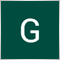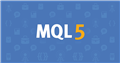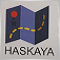22

Hey guys,

Nice to meet you all. I'm new to the forum

I want to create an Expert Advisor (Hedging EA) for the conditions below:

- 0.01 lot
- SL = 0, TP = 30 pips

If the price goes down to 30 pips below the open price of the Buy Order, it will:
--> Sell immediately:

- 0.03 lot

- SL = 30 pips, TP = 30 pips

AND

- SL = 60 pips, TP = 0 pips

2. The converse for Sell Order is true.

Below is my code of this EA

```//+------------------------------------------------------------------+
//|                                                 MA Crossover.mq4 |
//|                                                             Gary |
//|                                             https://www.mql5.com |
//+------------------------------------------------------------------+
#property strict

double magic = 1234;
//+------------------------------------------------------------------+
//| Expert initialization function                                   |
//+------------------------------------------------------------------+
int OnInit()
{
//---

//---
return(INIT_SUCCEEDED);
}
//+------------------------------------------------------------------+
//| Expert deinitialization function                                 |
//+------------------------------------------------------------------+
void OnDeinit(const int reason)
{
//---

}
//+------------------------------------------------------------------+
//| Expert tick function                                             |
//+------------------------------------------------------------------+
void OnTick()
{
//---
double EMA_21_Current = iMA(Symbol(), 0, 21, 0, 1, 0, 0);
double EMA_50_Current = iMA(Symbol(), 0, 50, 0, 1, 0, 0);
double EMA_200_Current = iMA(Symbol(), 0, 400, 0, MODE_EMA, 0, 0);

if(Hour()>=1 && EMA_21_Current > EMA_50_Current && EMA_50_Current > EMA_200_Current && Bid < EMA_21_Current && Bid > EMA_50_Current && OrdersTotal()==0)
{

for(int i =0;i<OrdersTotal();i++)
{
{
if(OrderSymbol()==Symbol() && OrderMagicNumber() == magic && OrderType()==OP_BUY)
{
SellTicket2 = OrderSend(Symbol(), OP_SELL, 0.03, Bid, 0, Bid+300*_Point, Bid-300*_Point, NULL, magic, 0, clrRed);
}
}
}

}

else if (Hour()>=1 && EMA_21_Current < EMA_50_Current && EMA_50_Current < EMA_200_Current && Bid > EMA_21_Current && Bid < EMA_50_Current && OrdersTotal()==0)
{
SellTicket = OrderSend(Symbol(), OP_SELL, 0.01, Bid, 0, Bid+350*_Point, Bid-350*_Point, NULL, magic, 0, clrBlueViolet);
for(int i =0;i<OrdersTotal();i++)
{
{
if(OrderSymbol()==Symbol() && OrderMagicNumber() == magic && OrderType()==OP_SELL)
{
Modify_Sell = OrderModify(SellTicket, OrderOpenPrice(),Bid-600*_Point, Bid+0*_Point, 0, clrBlack);
}
}
}

}

return;

}
//+------------------------------------------------------------------+

```

The problem of this EA is that it does not hedge by opening orders of opposite position. Can anyone look into it and help me with the code?Documentation on MQL5: Constants, Enumerations and Structures / Trade Constants / Trade Orders in DOM
• www.mql5.com
For equity securities, the Depth of Market window is available, where you can see the current Buy and Sell orders. Desired direction of a trade operation, required amount and requested price are specified for each order. To obtain information...549

Gary Hon:

Hey guys,

Nice to meet you all. I'm new to the forum

I want to create an Expert Advisor (Hedging EA) for the conditions below:

- 0.01 lot
- SL = 0, TP = 30 pips

If the price goes down to 30 pips below the open price of the Buy Order, it will:
--> Sell immediately:

- 0.03 lot

- SL = 30 pips, TP = 30 pips

AND

- SL = 60 pips, TP = 0 pips

2. The converse for Sell Order is true.

Below is my code of this EA

The problem of this EA is that it does not hedge by opening orders of opposite position. Can anyone look into it and help me with the code?

Hello,

you did not state the condition for hedging what you did is that after your initial Buy/Sell you checked for opened orders and if any found it should modify and open an opposite positions, that wont work.

you need to create a function that will read if your current Buy/Sell position is  30 pips negative from the open price then if true it should open an opposite position and modify the previous position.

this should solve it

i hope you found this helpful.2749

Gary Hon:

Hey guys,

Nice to meet you all. I'm new to the forum

I want to create an Expert Advisor (Hedging EA) for the conditions below:

- 0.01 lot
- SL = 0, TP = 30 pips

If the price goes down to 30 pips below the open price of the Buy Order, it will:
--> Sell immediately:

- 0.03 lot

- SL = 30 pips, TP = 30 pips

AND

- SL = 60 pips, TP = 0 pips

2. The converse for Sell Order is true.

Below is my code of this EA

The problem of this EA is that it does not hedge by opening orders of opposite position. Can anyone look into it and help me with the code?

It can be done simply this way. However, you should add more professional controls.

```//+------------------------------------------------------------------+
//|                                                 MA Crossover.mq4 |
//|                                                             Gary |
//|                                             https://www.mql5.com |
//+------------------------------------------------------------------+
#property strict

int magic = 1234;
int MagicMaster=99243;
int MagicSlave=99443;
input double LotMaster=0.10;
input double LotSlave=0.30;

//+------------------------------------------------------------------+
//| Expert initialization function                                   |
//+------------------------------------------------------------------+
int OnInit()
{
//---

//---
return(INIT_SUCCEEDED);
}
//+------------------------------------------------------------------+
//| Expert deinitialization function                                 |
//+------------------------------------------------------------------+
void OnDeinit(const int reason)
{
//---

}
//+------------------------------------------------------------------+
//| Expert tick function                                             |
//+------------------------------------------------------------------+
void OnTick()
{
//---
double EMA_21_Current = iMA(Symbol(), 0, 21, 0, 1, 0, 0);
double EMA_50_Current = iMA(Symbol(), 0, 50, 0, 1, 0, 0);
double EMA_200_Current = iMA(Symbol(), 0, 400, 0, MODE_EMA, 0, 0);
double Fark=0;
if(Hour()>=1 && EMA_21_Current > EMA_50_Current && EMA_50_Current > EMA_200_Current && Bid < EMA_21_Current && Bid > EMA_50_Current && OrdersTotal()==0)
{
//

}

else if (Hour()>=1 && EMA_21_Current < EMA_50_Current && EMA_50_Current < EMA_200_Current && Bid > EMA_21_Current && Bid < EMA_50_Current && OrdersTotal()==0)
{

SellTicket = OrderSend(Symbol(), OP_SELL, LotMaster, Bid, 0, 0,Bid-300*_Point, NULL, MagicMaster, 0, clrBlueViolet);

}
OnSeconOrderKontrol();
return;

}
//+------------------------------------------------------------------+
void OnSeconOrderKontrol()
{

double Fark=0;

for(int i =0;i<OrdersTotal();i++)
{
{
if(OrderSymbol()==Symbol() && OrderMagicNumber() == MagicMaster && OrderType()==OP_SELL && OrdersTotal()==1 )
{

if(Fark>320 && (MagicSlaveKontrol()==0))
{
Modify_Sell=OrderModify(OrderTicket(),OrderOpenPrice(),NormalizeDouble(OrderOpenPrice()+600*Point,Digits),0,0,clrRed);
//Modify_Sell = OrderModify(i, OrderOpenPrice(),Bid-600*_Point, 0, 0, clrBlack);
}
}

if(OrderSymbol()==Symbol() && OrderMagicNumber() == MagicMaster && OrderType()==OP_BUY && OrdersTotal()==1 )
{
Fark=(Bid-OrderOpenPrice())/Point;

if(Fark>320 && (MagicSlaveKontrol()==0))
{
Modify_Sell=OrderModify(OrderTicket(),OrderOpenPrice(),NormalizeDouble(OrderOpenPrice()-600*Point,Digits),0,0,clrRed);
//Modify_Sell = OrderModify(i, OrderOpenPrice(),Bid-600*_Point, 0, 0, clrBlack);
SellTicket2 = OrderSend(Symbol(), OP_SELL, LotSlave, Bid, 0, Bid+300*_Point, Bid-300*_Point, NULL, MagicSlave, 0, clrRed);
}
}
}
}

return;
}

int MagicSlaveKontrol()
{
int sonuc=0;
int total = OrdersTotal();
int ix;

for(ix=total-1;ix>=0;ix--)
{